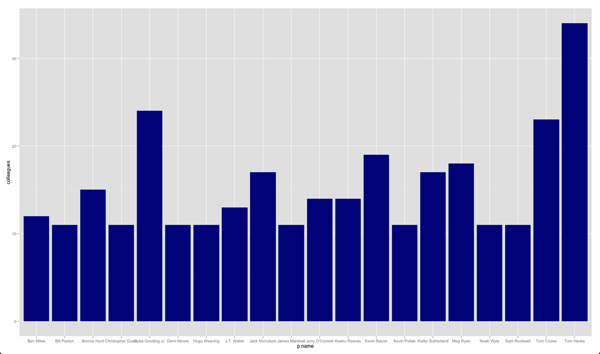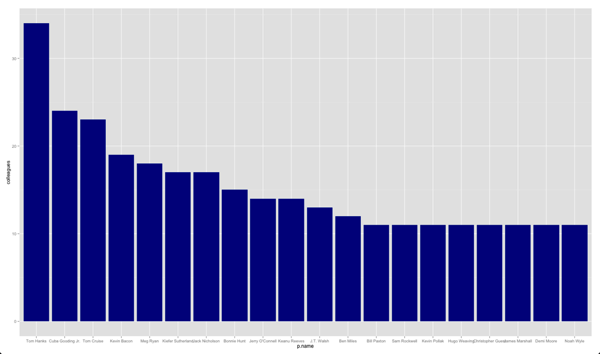# R/ggplot: Controlling X axis order

As part of a talk I gave at the Neo4j London meetup earlier this week I wanted to show how you could build a simple chart showing the number of friends that different actors had using the ggplot library.

I started out with the following code:

``````

ggplot(aes(x = p.name, y = colleagues), data = top) +
geom_bar(fill = "dark blue", stat = "identity")
``````

The friends CSV file is available as a gist if you want to reproduce the chart. This is how the chart renders:It's in a fairly arbitrary order when it would be quite cool if we could get the most popular people to show from left to right.

I had the people's names in the correct order in the data frame but annoyingly it was then sorting them into alphabetical order. Luckily I came across the by using the scale_x_discrete function which does exactly what I needed.

If we pass in the list of names to that function we get the chart we desire:

``````
ggplot(aes(x = p.name, y = colleagues), data = top) +
geom_bar(fill = "dark blue", stat = "identity") +
scale_x_discrete(limits= top\$p.name)
``````